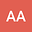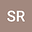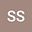Solutions of sum-type singular fractional q-integro-differential equation with $m$-point boundary value using quantum calculus
•••• +1
• Shahram Rezapour,
• Soheil Salahshour,
Mediterranean University of Reggio Calabria Department of Informatics Mathematics Electronics and Transport
Author ProfileShahram Rezapour
Author ProfileSoheil Salahshour
Bahcesehir Universitesi
Author ProfileBu Ali Sina University
Author Profile#### Peer review status:ACCEPTED

20 Jan 2020Submitted to Mathematical Methods in the Applied Sciences
25 Jan 2020Submission Checks Completed
25 Jan 2020Assigned to Editor
25 Jan 2020Reviewer(s) Assigned
01 Feb 2020Review(s) Completed, Editorial Evaluation Pending
02 Feb 2020Editorial Decision: Revise Major
13 Feb 2020Assigned to Editor
13 Feb 2020Submission Checks Completed
13 Feb 2020Reviewer(s) Assigned
15 Feb 2020Review(s) Completed, Editorial Evaluation Pending
12 Apr 2020Editorial Decision: Revise Major
In this study, we investigate the sum-type singular nonlinear fractional q-integro-differential $m$-point boundary value problem. The existence of positive solutions is obtained by the properties of the Green function, standard Caputo $q$-derivative, Riemann-Liouville fractional $q$-integral and the means of a fixed point theorem on a real Banach space $(\mathcal{X}, \|.\|)$ which has a partially order by using a cone $P \subset \mathcal{X}$. The proofs are based on solving the operator equation $\mathcal{O}_1 x + \mathcal{O}_2 x = x$ such that the operator $\mathcal{O}_1$, $\mathcal{O}_2$ are $r$-convex, sub-homogeneous, respectively and define on cone $P$. As applications, we provide an example illustrating the primary effects.